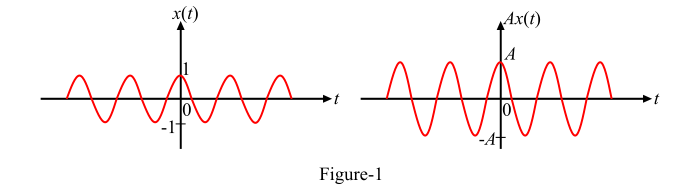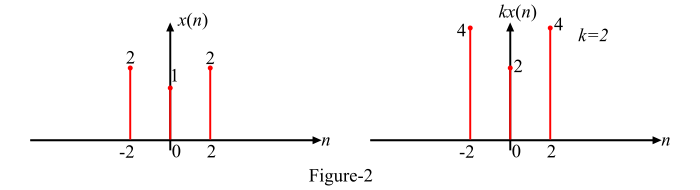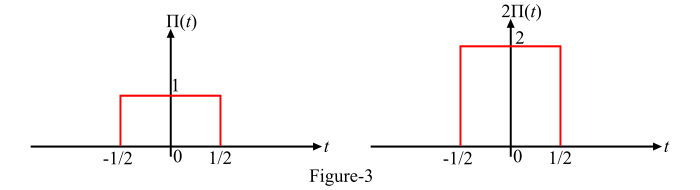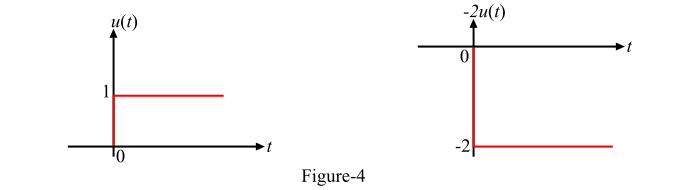# Signals and Systems: Amplitude Scaling of Signals

## What is Amplitude Scaling?

The process of rescaling the amplitude of a signal, i.e., the amplitude of the signal is either amplified or attenuated, is known as amplitude scaling. In the amplitude scaling operation on signals, the shape of the resulting signal remains the as that of the original signal but the amplitude is altered (i.e., increased or decreased).

## Amplitude Scaling of a Continuous-Time Signal

The amplitude scaling of a continuous time signal x(t) is defined as,

𝑦(𝑡) = 𝐴 𝑥(𝑡)

Where, A is a constant. If the value of A is greater than 1 (i.e., A > 1), the signal amplitude scaling is called the amplification of the signal while if A < 1, then the scaling is called the attenuation of the signal.

Figure-1 shows an arbitrary continuous-time signal x(t) and its amplitude scaled version y(t).## Amplitude Scaling of a Discrete-Time Signal

The amplitude scaling of a discrete time sequence x(n) is defined as,

𝑦(𝑛) = 𝑘 𝑥(𝑛)

Where, k is a constant. If k > 1, the scaling is called amplification of the signal, while if k < 1, the scaling is called attenuation of the signal.

An arbitrary discrete time sequence x(n) and its scaled version y(n) are shown in Figure-2.## Numerical Example

Sketch the following continuous-time signal x(t) −

• 𝑥(𝑡) = Π(𝑡); 𝑦(𝑡) = 2 Π(𝑡)

• 𝑥(𝑡) = 𝑢(𝑡); 𝑦(𝑡) = −2𝑢(𝑡)

### Solution

• The given signal is a unit rect function, i.e.,

𝑥(𝑡) = Π(𝑡)

The amplitude scaled signal [𝑦(𝑡) = 2 Π(𝑡)] of the given signal is obtained by multiplying the amplitude of x(t) by 2 as shown in Figure-3. Here, it is to be noted that the value of scaling factor more than 1 (i.e., 2), hence the resulting signal will be an amplified signal.• The given signal is a unit step function, i.e.,

𝑥(𝑡) = 𝑢(𝑡)

Here, in order to obtain the scaled signal [𝑦(𝑡) = −2𝑢(𝑡)], the amplitude of the signal x(t) needs to be multiplied by (-2). Figure-4 shows the signals x(t) and y(t).